Dot

Mathematica computes the dot product of two vectors exactly as we do in Linear Algebra. In the next cell we get the Dot product of two 3-D vectors.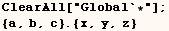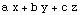In the next cell we try to compute the Dot product of things that aren't litsts, and we see it doesn't work.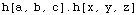One of the advantages of Inner is that it can do what we tried to do in the previous cell as we see with the next cell.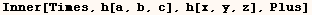Below we see that matrix multiplication is also implemented using Dot.

The Dot product of a vector and a matrix

We can compute the Dot product of a vector and a matrix.  In the next cell we get the Dot product of (vector A) with each column of (matrix B).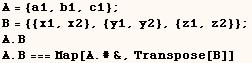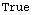However, in the next example we get the Dot product of each row of (matrix A) with (vector B).The Dot product of two matrices

When Mathematica computes the Dot product of matrices you essentially get the product of the matrices as defined in Linear Algebra. Hence if A is a (m × n) matrix and B is a (n × j) matrix then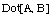is a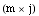matrix. A generic example is given in the next cell.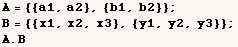The Dot product of two tensors

Mathematica can compute the Dot product of tensors with compatible dimensions. In the next cell the Dot product of tensors A and B is computed.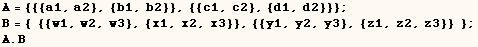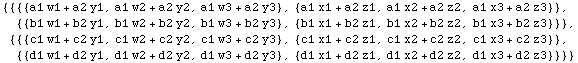The next cell helps explain how parts of the dot product above are computed.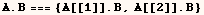In the cell above A[] is a matrix, but B is a tensor. The next cell shows how parts of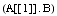are computed. The parts of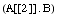are computed in a similar manner. All the Dot products below involve vectors and matrices and are computed as explained above.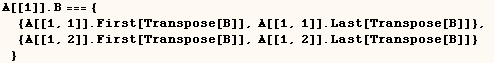The Dot product of three or more arguments

Mathematica can also compute Dot[A1, A2, A3,  ... ]  provided  (A1, A2, A3, ...) have suitable dimensions.
Dot[A1, A2, A3 ]  can be considered a concise way or writing Dot[A1, Dot[A2, A3] ] or
Dot[Dot[A1, A2], A3]].  An example of the Dot product of three arguments is given in the next cell.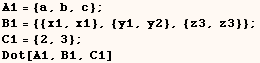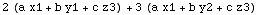Created by Mathematica  (May 16, 2004)

Back to Ted’s Tricks index page